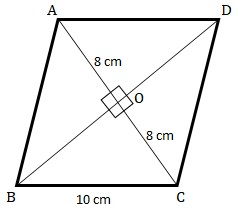# Each side of a rhombus is 10 cm. If one of its diagonals is 16 cm find the length of the other diagonal.

Given:

Length of each side of the rhombs$=10\ cm$. Length of one of the diagonals$=16\ cm$.

To do:

We have to find the length of the other diagonal.

Solution:Let ABCD be the rhombus in which O is the intersecting point of the diagonals.

We know that,

Diagonals of a rhombus bisect each other at right angles.

Therefore,

$\angle BOC=90^o$, $BC=10\ cm$ and $OC=8\ cm$

$\triangle BOC$ is a right-angle triangle.

This implies, using Pythagoras theorem,

$BC^2=BO^2+OC^2$

$(10)^2=(8)^2+OC^2$

$BO^2=(100-64)\ cm^2$

$BO^2=36\ cm^2$

$BO=\sqrt{36}\ cm$

$BO=6\ cm$

$BD=2(BO)=2(6)\ cm$

$BD=12\ cm$

The length of the other diagonal is 12 cm.

Updated on: 10-Oct-2022

17 Views